# C Program to Count the Number of Vowels, Consonants and so on

In this tutorial you will learn about the C Program to Count the Number of Vowels, Consonants and so on and its application with practical example.

## C Program to Count the Number of Vowels, Consonants and so on

In this tutorial, we will learn to create a C program that will Count the Number of Vowels, Consonants, and so on in C programming

## Prerequisites

Before starting with this tutorial we assume that you are best aware of the following C programming topics:

• Operators in C Programming.
• Basic Input and Output function in C Programming.
• Basic C programming.
• For loop in c programming.

## Count the Number of Vowels, Consonants, and so on

As we all know the String is a collection of character data types. In strings, only one variable is declared which can store multiple values. First will take the line of string from the user. Then will take the number of elements in the string, Now we will filter the string using the c programming.

With the help of this program, we can Count the Number of Vowels, Consonants, and so on.

## Output:-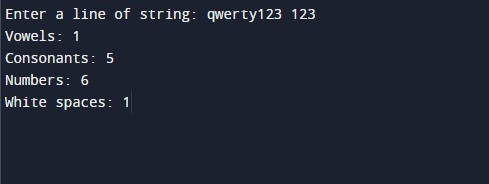In the above program, we have first initialized the required variable.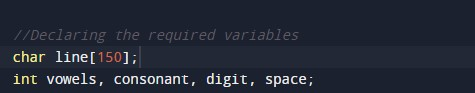• line = it will hold the string value.
• vowels = it will hold the integer value for vowels.
• consonant  = it will hold the integer value for consonants.
• digit= it will hold the integer value for numbers.
• space= it will hold the integer value for spaces.

Input strings from the user.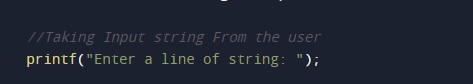Assigning blank value to all to prevent garbage value.Calculating the size of the string.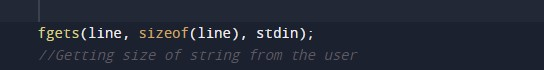Filtering vowels from the string.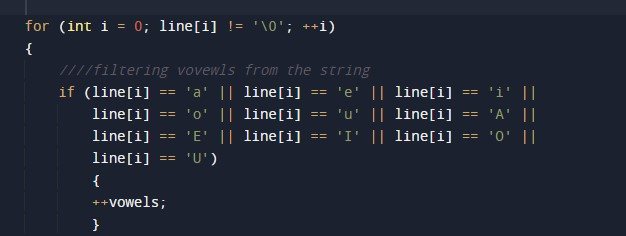consonants filtering from the string.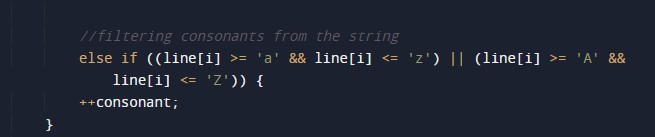filtering numbers from the string.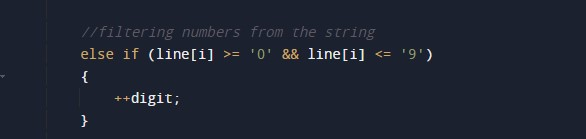filtering white spaces from the string.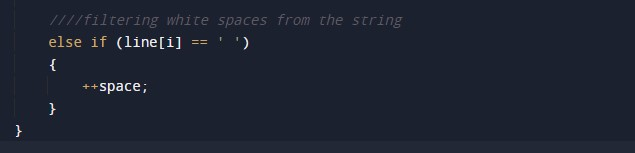Printing output.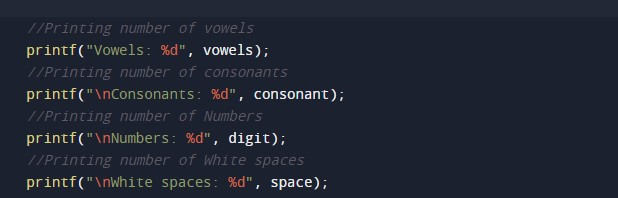In this tutorial we have learn about the C Program to Count the Number of Vowels, Consonants and so on and its application with practical example. I hope you will like this tutorial.